Home > CALC > Chapter Ch7 > Lesson 7.1.3 > Problem7-34

7-34.
1.Without a calculator, determine the following limits. Homework Help ✎

1.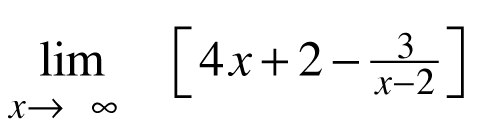2.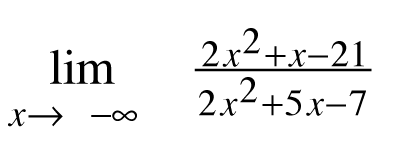3.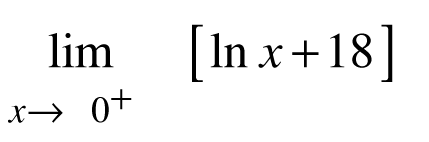4.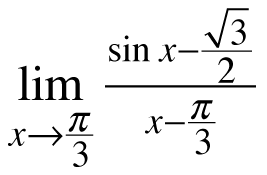2.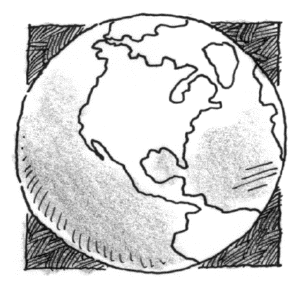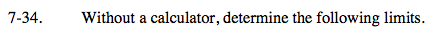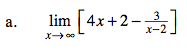If you took the limit of each term separately, would any of those limits = 0?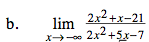Check the end behavior of the graph by comparing the highest powers from both the numerator and the denominator.

$\lim_{x\rightarrow -\infty }\frac{2x^{2}}{2x^{2}}=\lim_{x\rightarrow -\infty }1=\text{ \_\_\_\_ }$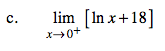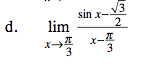This is Ana's Definition of the Derivative;
Find f '(a).

$f'(a)=\lim_{x\rightarrow a}\frac{f(x)-f(a)}{x-a}$